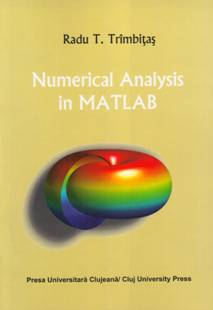# Numerical Analysis in MATLAB

## ISBN 978-973-610-841-9

458+16  pages

Contents

1. Introduction to MATLAB
2. MATLAB Graphics
3. Errors and Floating Point Arithmetic

4.      Numerical Solution of Linear Algebraic Systems

5.      Function Approximation

6.      Linear Functional Approximation

7.      Numerical Solution of Nonlinear Equations

8.      Eigenvalues and Eigenvectors

9.      Numerical Solution of Ordinary Differential Equations

10.  Multivariate Approximation

Bibliography

Index